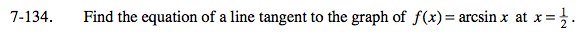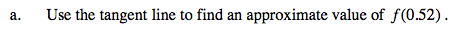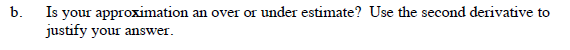### Home > CALC > Chapter 7 > Lesson 7.3.3 > Problem7-134

7-134.
1. Find the equation of a line tangent to the graph of f(x) = arcsin x at x =. Homework Help ✎

1. Use the tangent line to find an approximate value of f(0.52).

2. Is your approximation an over or under estimate? Use the second derivative to justify your answer.Because y = arcsin(0.52) is hard to evaluate, the tangent line at x = 0.52 will give a decent approximation of f(0.52).and that will indicate if the tangent line is above or below the graph.